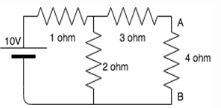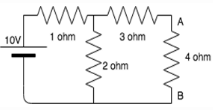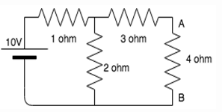# Test: Thevenin’s Theorem

## 10 Questions MCQ Test Network Theory (Electric Circuits) | Test: Thevenin’s Theorem

Description
Attempt Test: Thevenin’s Theorem | 10 questions in 10 minutes | Mock test for Electrical Engineering (EE) preparation | Free important questions MCQ to study Network Theory (Electric Circuits) for Electrical Engineering (EE) Exam | Download free PDF with solutions
QUESTION: 1

### Calculate the Thevenin resistance across the terminal AB for the following circuit.​Solution:

Thevenin resistance is found by opening the circuit between the specified terminal and shorting all voltage sources.
When the 10V source is shorted, we get:
Rth=(1||2)+3=3.67 ohm.

QUESTION: 2

### Calculate Vth for the given circuit.​Solution:

Thevenin resistance is found by opening the circuit between the specified terminal and shorting all voltage sources.
When the 10V source is shorted, we get:
Rth=(1||2)+3=3.67 ohm.
Vth is calculated by opening the specified terminal.
Using voltage divider, Vth= 2*10/(2+1)=6.67V.

QUESTION: 3

### Calculate the current across the 4 ohm resistor.​Solution:

Thevenin resistance is found by opening the circuit between the specified terminal and shorting all voltage sources.
When the 10V source is shorted, we get:
Rth=(1||2)+3=3.67 ohm.
Vth is calculated by opening the specified terminal.
Using voltage divider, Vth= 2*10/(2+1)=6.67V.
On drawing the Thevenin equivalent circuit, we get Rth, 4 ohm and Vth in series.
Applying ohm’s law, I=Vth/(4+Rth)= 0.86A.

QUESTION: 4

The Thevenin voltage is the__________

Solution:

Thevenin voltage is the open circuit voltage. It is the voltage across the specified terminals. It is not the short circuit voltage because short circuit voltage is equal to zero.

QUESTION: 5

Thevenin resistance is found by ________

Solution:

Current sources have infinite internal resistance hence behave like an open circuit whereas ideal voltage sources have 0 internal resistance hence behave as a short circuit.

QUESTION: 6

Thevenin’s theorem is true for __________

Solution:

Thevenin’s theorem works for only linear circuit elements and not non-linear ones such as BJT, semiconductors etc.

QUESTION: 7

In Thevenin’s theorem Vth is__________

Solution:

Thevenin’s theorem states that a combination of voltage sources, current sources and resistors is equivalent to a single voltage source V and a single series resistor R.

QUESTION: 8

Vth is found across the ____________ terminals of the network.

Solution:

According to Thevenin’s theorem, Vth is found across the output terminals of a network and not the input terminals.

QUESTION: 9

Which of the following is also known as the dual of Thevenin’s theorem?

Solution:

Norton’s theorem is also known as the dual of Thevenin’s theorem because in Norton’s theorem we find short circuit current which is the dual of open circuit voltage-what we find in Thevenin’s theorem.

QUESTION: 10

Can we use Thevinin’s theorem on a circuit containing a BJT?

Solution:

We can use Thevenin’s theorem only for linear networks. BJT is a non-linear network hence we cannot apply Thevenin’s theorem for it.Use Code STAYHOME200 and get INR 200 additional OFF Use Coupon Code